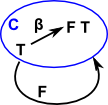# Maths - Category Theory - F-Algebra and F-Coalgebra

I assume the 'F' in 'F-Algebra' stands for functor?

 An algebra is defined by: F a -> a where: a is an object in cat, for example, 'a' could be Int or Bool. This is known as the carrier type. F is a endofunctor which forms expressions. in Idris: ```interface Algebra f a = f a -> a```

Some explanation is required to make sense of this.

## Signature of an Algebra

We can think of a binary operation as taking two numbers and returning another number. So
a = b * c
is a mapping
(b,c) -> a

Since a,b & c are all of the same type (such as real numbers) we can show the type signature as:

T -> T²

where

• T² = T×T
• × = the cartesian product

Note: this constructs the signature 'F' then goes in the opposite direction and allows us to evaluate expressions.

So in the case of numbers, T is the set of all numbers so T² is the cartesian product of these sets.

So far we have just looked at one operation in an algebra, lets now look a whole algebra, an example might be groups. A group has one binary operation (multiplication), one unary operation (inverse) and one identity element. The binary operation has a domain type of T², the unary operation has a domain type of T and the identity has a domain type of 1. We can therefore give the signature functor F of an algebra as a polynomial:

F : T -> T² + T + 1

where

• T² = T×T
• × = the cartesian product
• + = disjoint union
• 1 = identity element (final object)

So in the reverse direction we can get functors like these:

 0
1 -> T
 0 1 2 3 4
 0 1 2 3 4
T -> T
 0 1 2 3 4
 (0,0) (0,1) (0,2) (0,3) (0,4) (1,0) (1,1) (1,2) (1,3) (1,4) (2,0) (2,1) (2,2) (2,3) (2,4) (3,0) (3,1) (3,2) (3,3) (3,4) (4,0) (4,1) (4,2) (4,3) (4,4)
T² -> T
 0 1 2 3 4

## F-Algebra & F-Coalgebra

The functor 'F' that we have defined above is an endofunctor to/from a category C which contains the type T and its powers to allow the following:

F-Algebra F-Coalgebra

An F-Algebra consists of a pair: (T, α) which is a type and a function α which is defined as:

α : F T -> T

An F-Coalgebra consists of a pair: (T, β) which is a type and a function β which is defined as:

β : T -> F TWhere I can, I have put links to Amazon for books that are relevant to the subject, click on the appropriate country flag to get more details of the book or to buy it from them.The Princeton Companion to Mathematics - This is a big book that attempts to give a wide overview of the whole of mathematics, inevitably there are many things missing, but it gives a good insight into the history, concepts, branches, theorems and wider perspective of mathematics. It is well written and, if you are interested in maths, this is the type of book where you can open a page at random and find something interesting to read. To some extent it can be used as a reference book, although it doesn't have tables of formula for trig functions and so on, but where it is most useful is when you want to read about various topics to find out which topics are interesting and relevant to you.